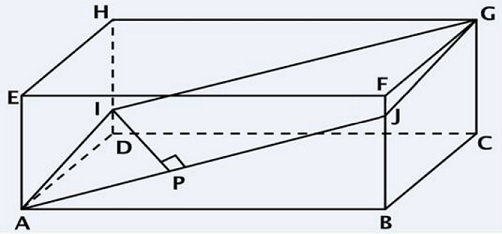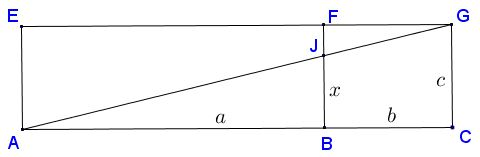# Optimization in Parallelepiped

### Problem

The following problem by Dan Sitaru and Leo Giugiuc has been posted together with a solution (Solution 1) by Dan Sitaru at the CutTheKnotMath facebook page:

Given $a,b,c\gt 0.$ Find minimum and maximum of $f(x)=\sqrt{a^2+x^2}+\sqrt{b^2+(c-x)^2}$ on interval $[0,c].$

### Solution 1

Consider a parallelepiped (the diagram below), with $AB=a,$ $BC=b,\;$ and $BF=c.$ Let $x=BJ.$The diagram reveals several relationships between the elements:

$AJ\le AF,\text{ i.e., } \sqrt{a^2+x^2}\le\sqrt{a^2+c^2}\\ GJ\le GB=\sqrt{b^2+c^2},\\ FJ=c-x,\\ GJ\le GB,\text{ i.e.,} =\sqrt{b^2+(c-x)^2}\le \sqrt{b^2+c^2}.$

If $AF+FG=\Omega_1$ and $AB+BG=\Omega_2$ then, $\text{max} f(x)=\max\{\Omega_1,\Omega_2\}.$

Consider just two faces of the parallelepiped:$f(x)$ is exactly the path $AJ+JG$ which attains it minimum value only of $AJG$ is a straight line. This happens exactly when $\displaystyle\frac{x}{c}=\frac{a}{a+b},$ so that $\displaystyle x=\frac{ac}{a+b}.$

Note that the algebraic formulation conceals an old problem that requires from a spider sitting in the vertex $A$ to reach the fly in the vertex $G$ in the shortest way possible. The second solution reveals another geometric interpretation.

### Solution 2

This solution is due to Francisco Javier García Capitán and it draws on the famous Heron's problem.

We observe that $f(x)$ is the distance $AX+BX$ where $A=(0,a),\;$ $B=(c,b),\;$ and $X=(0,x).\;$Since $AX+BX=AX+B'X,\;$ where $B'$ is the reflection of $B$ in the $x$ axis, $f(x)$ will be minimum when $A,\;$ $X\,\;$ and $B'\;$ are collinear.

To find $x$ we can use similar triangles $AOX\;$ and $B'MX\;$ (with $M=(c,0)):$

$\displaystyle \frac{OX}{OA}=\frac{MX}{MB'},$ or $\displaystyle\frac{x}{a}=\frac{c-x}{b},$

from which $\displaystyle a=\frac{ac}{a+b}.$

### A Sample of Optimization Problems II

• Mathematicians Like to Optimize
• Building a Bridge
• Building Bridges
• Optimization Problem in Acute Angle
•